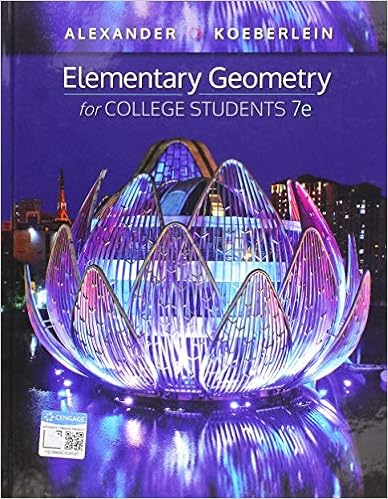# Exam 2 1997 - 110.201 LINEAR ALGEBRA 5 November 1997 Second...

• Test Prep
• linda.z.cho
• 4

This preview shows page 1 - 2 out of 4 pages.

##### We have textbook solutions for you!
The document you are viewing contains questions related to this textbook.The document you are viewing contains questions related to this textbook.
Chapter 1 / Exercise 4
Elementary Geometry for College Students
Alexander/KoeberleinExpert Verified
110.201 LINEAR ALGEBRA5 November 1997Second Examination50 minutes. Closed book. No notes. 80 points, 20 per question.Partial credit may be available, but only if you show your working.HINT: There are many opportunities to check your answers.Leave expressions such as2andcos-1(1/3)alone; do not evaluate them.No answer should contain an unexpanded determinant.Begin each of the four questions on a new page and number it clearly in the margin.1.A triangle inR3has the verticesA= (1,0,1),B= (3,2,3) andC= (2,4,3).(a) Find the angle atA.(b) Find (by any method) an equation for the plane that contains the triangleABC.2.A right-angled triangle inR4has the verticesP= (2,5,2,2),Q= (1,1,3,3) andR= (0,3,1,2).(a) At which vertex is the right angle? Explain how you know.(b) Find the lengths of all three sides.(c) Verify Pythagoras’s Theorem for this triangle.3.(a) Find the matrix of the linear transformation of the planeR2that rotates everyvectorclockwisethrough an angle of 60 degrees.(Recall that cos(π/3) = 1/2 andthat sin(π/3) =3/2.)(b) Find the matrix of the dilation ofR2that makes every vector three times aslong (and leaves its direction unchanged).
##### We have textbook solutions for you!
The document you are viewing contains questions related to this textbook.The document you are viewing contains questions related to this textbook.
Chapter 1 / Exercise 4
Elementary Geometry for College Students
Alexander/KoeberleinExpert Verified
•••